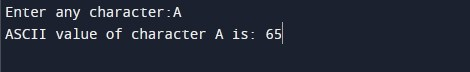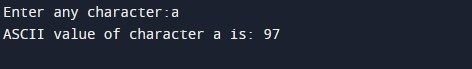C Program to Print ASCII Value of a Character

In this tutorial you will learn about the C Program to Print ASCII Value of a Character and its application with practical example.

In this tutorial, we will learn to create a program to print ASCII value of a character in C programming.

Prerequisites

Before starting with this tutorial we assume that you are best aware of the following C programming topics:

• C Data Types
• C Variables
• C Input Output

What Is ASCII value of a Character?

ASCII value is typically a numeric representation of the English characters (an integer number between 0 and 127) rather than that character itself. This integer value is referred as ASCII code of the character. For example, the ASCII value of uppercase ‘A’ is 65. This means when you assign ‘A’ to a character variable, 65 is stored in the variable rather than ‘A’ itself.

Program to Print ASCII Value of a Character

In this program we will print ASCII value of a character entered by the user. We would first declared and initialized the required variables. Next, we would prompt user to input a character. Later in the program we will display the ASCII value of the user specified character.

Output 1:-Output 2:-In the above program, we have first declared and initialized a set variables required in the program.

• ch = it holds the character value entered by user

In the next statement user will be prompted to enter a character values which will be assigned to variable ‘ch’. Next, we will be displaying the ASCII value of the character using integer format specifier(%d) in printf statement.

In this tutorial we have learn about the C Program to Print ASCII Value of a Character and its application with practical example. I hope you will like this tutorial.